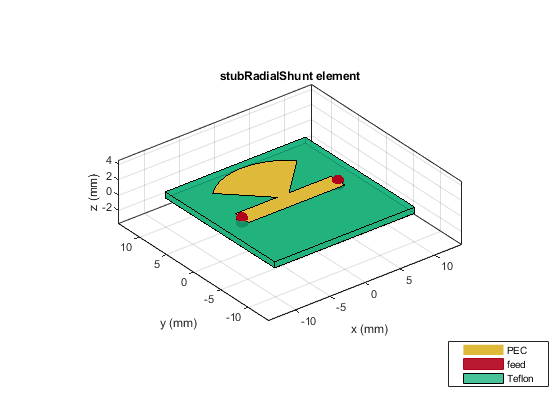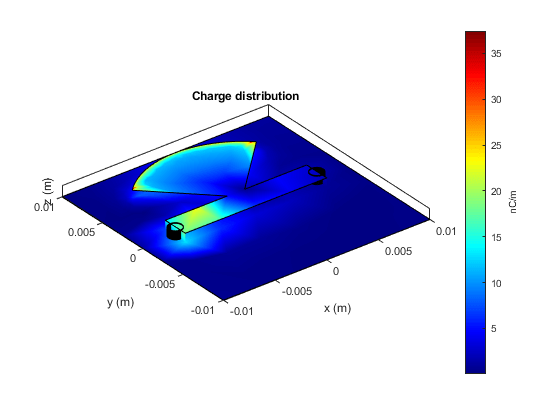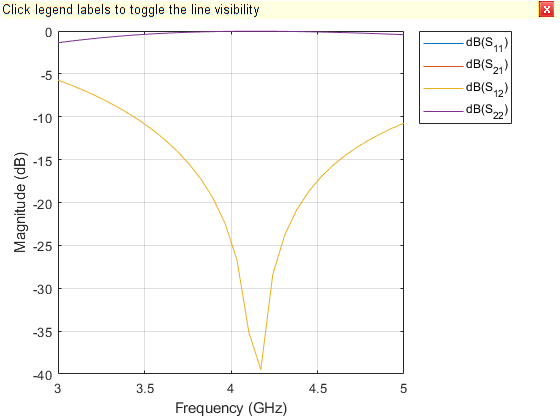# Shunt Radial Stub - Visualize and Analyze

This example shows you how to create, visualize, and analyze a radial stub shunt on the X-Y plane.

Create a shunt radial stub with default properties.

`stub = stubRadialShunt`
```stub = stubRadialShunt with properties: StubType: 'Single' OuterRadius: 0.0085 InnerRadius: 0.0012 Angle: 90 PortLineLength: 0.0137 PortLineWidth: 0.0025 Height: 8.0000e-04 GroundPlaneLength: 0.0200 GroundPlaneWidth: 0.0200 Substrate: [1x1 dielectric] Conductor: [1x1 metal] ```

View the stub.

`show(stub)`Plot the charge distribution at 5 GHz.

`charge(stub, 5e9)`Plot the current distribution at 5 GHz.

```figure current(stub, 5e9)```Calculate and plot the s-parameters.

```spar = sparameters(stub,linspace(3e9,5e9,30)); rfplot(spar)```